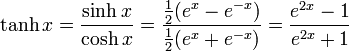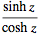getcarter

## Homework Statement

How to verify this trigonometric equation? tanh z/2?
verify that:

tanh (z/2) = [sinh x + i sin y] / [cosh x + cos y]

z is a complex number.

How can i verify this equation?

## Homework Equations# sin(x + i y) = sin(x) cosh(y) + i cos(x) sinh(y).
# cos(x + i y) = cos(x) cosh(y) - i sin(x) sinh(y).
# tan(x + i y) = (tan(x) + i tanh(y)) / (1 - i tan(x) tanh(y)).
# cot(x + i y) = (cot(x) coth(y) - i) / (i cot(x) + coth(y)).

## The Attempt at a Solution

i have tried to solve from right hand side but it didn't work, then i have simplified the equation tanh(z/2) = e^z-1 / e^z+1

if you help me, i will be appreciate.
thanx a lot.

Homework Helper
tanh(z/2)=(e^z-1)/(e^z+1) is an ok place to start. Now substitute z=x+iy and multiply numerator and denominator by the conjugate of (e^z+1). Multiply everything out. Where does that take you? What you eventually need is cosh(ix)=cos(x) and sinh(ix)=i*sin(x) for x real.

getcarter
thank u very much.

ok i have substitued equation but after i don't know how can i simplify.

Homework Helper
What did you get? Did you multiply by the conjugate and collect terms? It works for me.

getcarter
yes i did sir.

e^2(x+iy)-2e^(x+iy)+1 / e^2(x+iy)-1

did u mean complex conjugate ? or normal conjugate?

Homework Helper
The conjugate of the denominator is (e^(x-iy)+1). It looks like you multiplied by (e^(x+iy)-1). Try the first way.

Homework Helper
yes i did sir.

e^2(x+iy)-2e^(x+iy)+1 / e^2(x+iy)-1

did u mean complex conjugate ? or normal conjugate?

Complex. BTW, I suggested that because the denominator of the expression you are supposed to get is real. See why that's a clue?

getcarter
ok result is

e^2x + e^(x+iy) - e^(x-iy)-1 / e^2x + e^(x+iy) + e^(x-iy)

getcarter
than i don't know how can simplify this eq.

sorry, i am taking your time.